Win in raffle

The raffle tickets were sold 200, 5 of which were winning. What is the probability that Peter, who bought one ticket will win?

Result

p =  12.005 %

Solution:Leave us a comment of example and its solution (i.e. if it is still somewhat unclear...):Be the first to comment!To solve this example are needed these knowledge from mathematics:

Our percentage calculator will help you quickly calculate various typical tasks with percentages. Would you like to compute count of combinations?

Next similar examples:

1. Probability - tickets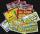What is the probability that when you have 25 tickets in 5000 that you wins the first (one) prize?
2. CardsSuppose that are three cards in the hats. One is red on both sides, one of which is black on both sides, and a third one side red and the second black. We are pulled out of a hat randomly one card and we see that one side of it is red. What is the probabi
3. Probability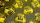What are the chances that the lottery, in which the numbers are drawn 5 of 50 you win the first prize?
4. Lathe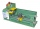95% of the components manufactured on the lathe comply with the standard, of which 80% of the components are first-class. How likely can we expect a manufactured part to be first class?
5. ThrowWe throw 2 times with 2 dices. What is the probability that the first roll will fall more than sum of 9 and the second throw have sum 3 or does not have the sum 4?
6. Medicine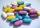We test medicine on 6 patients. For all drug doesn't work. If the drug success rate of 20%, what is the probability that medicine does not work?
7. The diceWhat is the probability of events that if we throw a dice is rolled less than 6?
8. Sum 10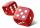What is the probability that two dice throw twice in a row will result the sum of 10?
9. Green - Red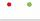We have 5 bags. Each consist one green and 2 red balls. From each we pull just one ball. What is the probability that we doesn't pull any green ball?
10. BallsThe urn is 8 white and 6 black balls. We pull 4 randomly balls. What is the probability that among them will be two white?
11. In the orchardIn the orchard, they planted 25 apple trees, 20 pears, 15 plums and 40 marbles. A strong late frost, however, destroyed a fifth of all new trees. Unfortunately, it was all the trees of one kind of fruit. What is the probability that the plums have died out
12. Theorem proveWe want to prove the sentence: If the natural number n is divisible by six, then n is divisible by three. From what assumption we started?
13. CandiesIn the box are 12 candies that look the same. Three of them are filled with nougat, five by nuts, four by cream. At least how many candies must Ivan choose to satisfy itself that the selection of two with the same filling? ?
14. ShootersIn army regiment are six shooters. The first shooter target hit with a probability of 49%, next with 75%, 41%, 20%, 34%, 63%. Calculate the probability of target hit when shooting all at once.
15. Profit gainIf 5% more is gained by selling an article for Rs. 350 than by selling it for Rs. 340, the cost of the article is:
16. Test pointsIf you earned 80% of the possible 40 points, how many points did you miss to get 100%?
17. BorrowingI borrow 25,000 to 6.9% p.a.. I pay 500 per month. How much will I pay and for how long?